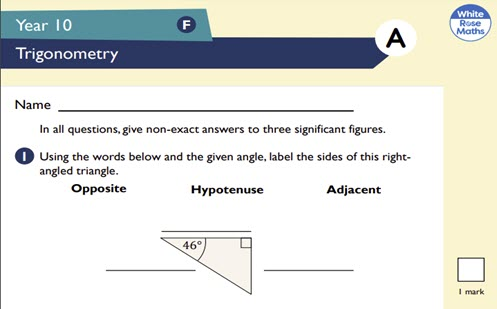# Assessment: Year 9 trigonometry

Use this two-page worksheet to assess a student's understanding of trigonometry. Each question has an allocated mark.

Year level(s) Year 9
Audience Teacher
Teaching strategies and pedagogical approaches Feedback
Keywords Trigonometry

## Curriculum alignment

Strand and focus Space
Topics Pythagoras and trigonometry
AC: Mathematics (V9.0) content descriptions
AC9M9SP02
Apply the enlargement transformation to shapes and objects using dynamic geometry software as appropriate; identify and explain aspects that remain the same and those that change

AC9M9M03

Solve spatial problems, applying angle properties, scale, similarity, Pythagoras' theorem and trigonometry in right-angled triangles

Numeracy progression Understanding geometric properties (P7)
Proportional thinking (P7)
Understanding units of measurement (P10)